# MATLAB Assignment 2

## Math 473 Spring 2021

This document is called a live script. It combines MATLAB code, formatted text (like a word processor) and the outputs from the code (including graphs and the results of printing statements) into an integrated document. This is a great format for turning in homework. Feel free to make a copy of this document, and use my codes as the basis for your homework. Just be sure to delete any of the words are code that would not form part of your answer, things like this paragraph. After you write and run the code, click the triangle beneath the "Save" button at the top-left of this window and save the result to a PDF to hand it in.
You learned in class the Euler method for approximating a solution to the differential equationGiven an initial condition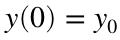, the method gives a sequence of approximations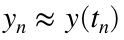using the recursion scheme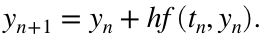where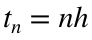and h is the time step chosen by the user.

## Warmup

We could use a simpler recursion to define a sequence of valuesWe know the exact solution to this recurrence isso we plot this as well;
N = 10;
n=0:N;
x = zeros(1,N+1);
x(1) = 0;
for j=1:N
x(j+1) = x(j) + j;
end
xExact=n.*(n+1)/2; % note the use of .* to multiply arrays!
plot(n,x,'o',n,xExact,'x')
xlabel('n');ylabel('y')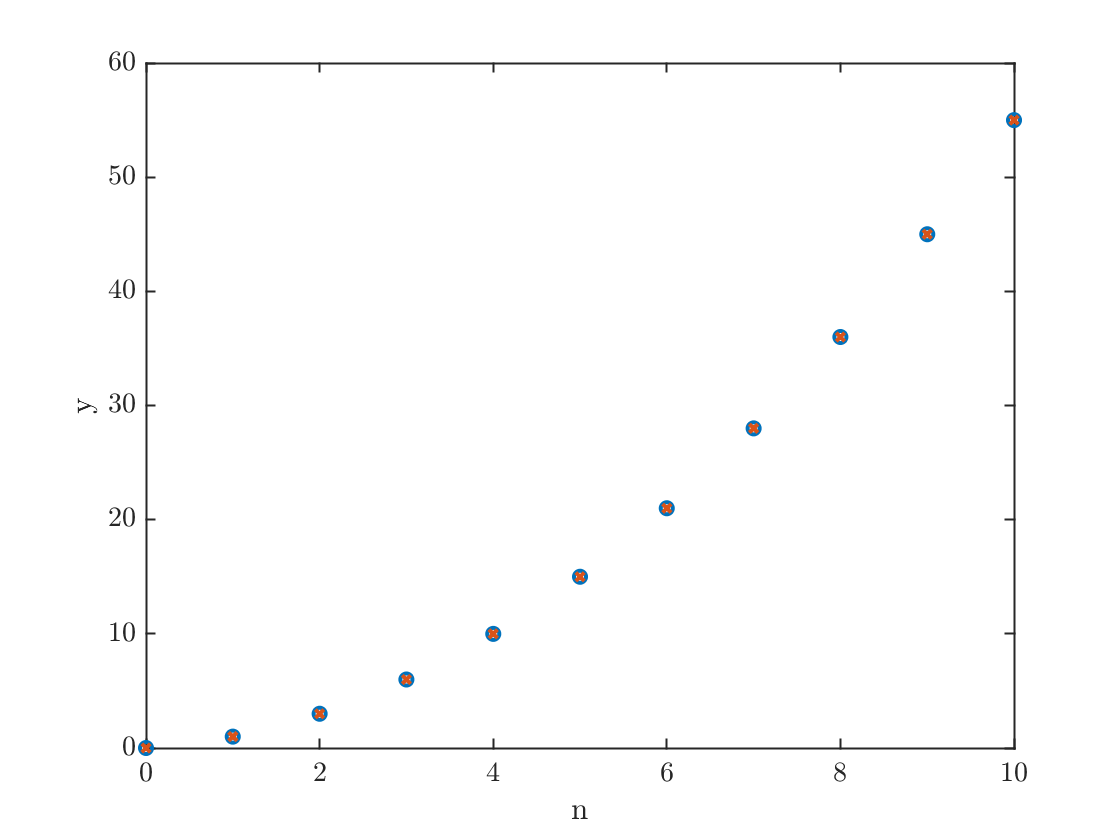## The Euler method

Now we're ready for the real thing. Let's solve the problemThis has exact solution (which is easy to find because the ODE is separable)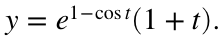tFinal = 2*pi;
N = 100;
h=tFinal/N;
t=linspace(0,tFinal,N+1); % type 'help linspace' to see how this works
x=zeros(1,N+1);
xExact=exp(1-cos(t)).*(1+t);
I want to point out the syntax used to define yExact in the above line. In particular the .* notation which is used for elementwise multiplication, since exp(1-cos(t)) and (1+t) are each vectors. You will need this to form the exact solution below, you will also need to use the .^ notation to compute an elementwise power.
x(1) = 1; % setting the initial condition
for n=1:N
x(n+1) = x(n) + h * (sin(t(n))+1/(1+t(n)))*x(n);
end
plot(t,x,t,xExact,'--'); xlabel('t'); ylabel('y'); title('Look, ma! I solved an ODE in MATLAB!');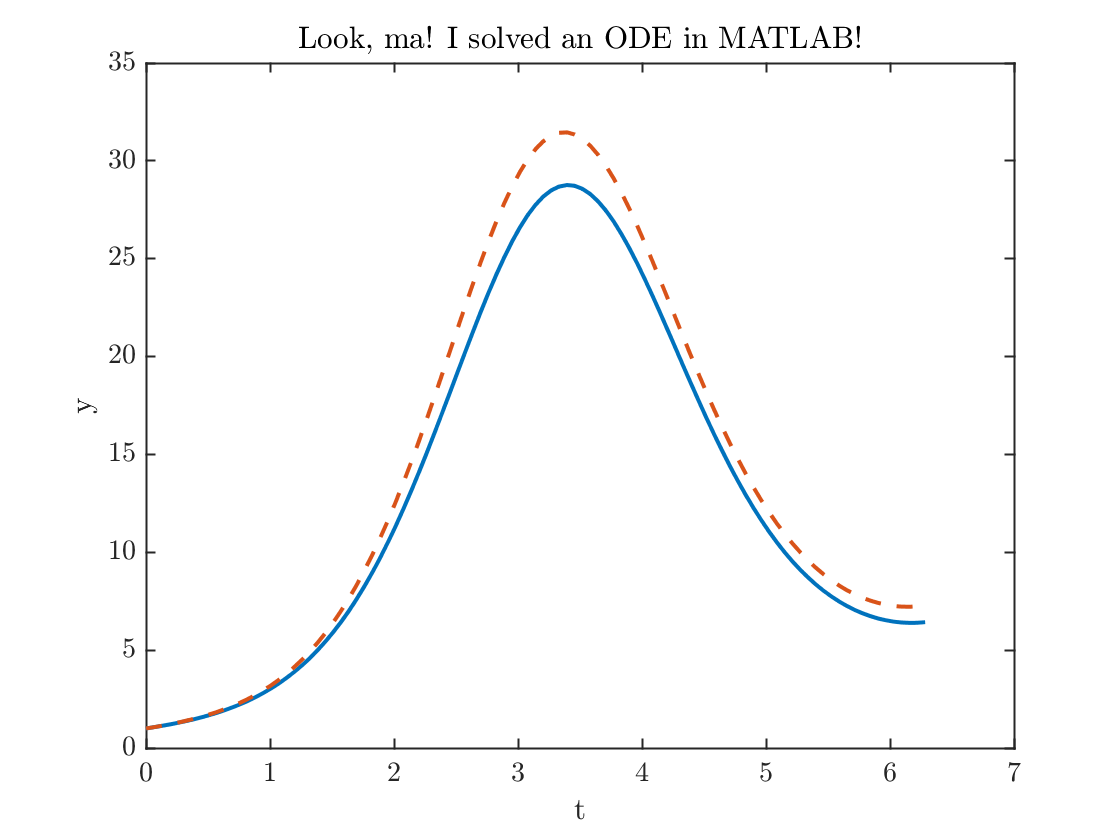error100= max(abs(x-xExact));
fprintf('The maximum error = %0.2f.\n',error100)
The maximum error = 2.85.
Note I used the max function to evaluate the error.
That error is a little bit larger than I'd like, let's double the number of points and try again
N = 200;
h=tFinal/N;
t=linspace(0,tFinal,N+1); % type 'help linspace' to see how this works
x=zeros(1,N+1);
xExact=exp(1-cos(t)).*(1+t);
x(1) = 1; % setting the initial condition
for n=1:N
x(n+1) = x(n) + h * (sin(t(n))+1/(1+t(n)))*x(n);
end
plot(t,x,t,xExact,'--')
xlabel('t'); ylabel('y'); title('Look, ma! I solved it even better!');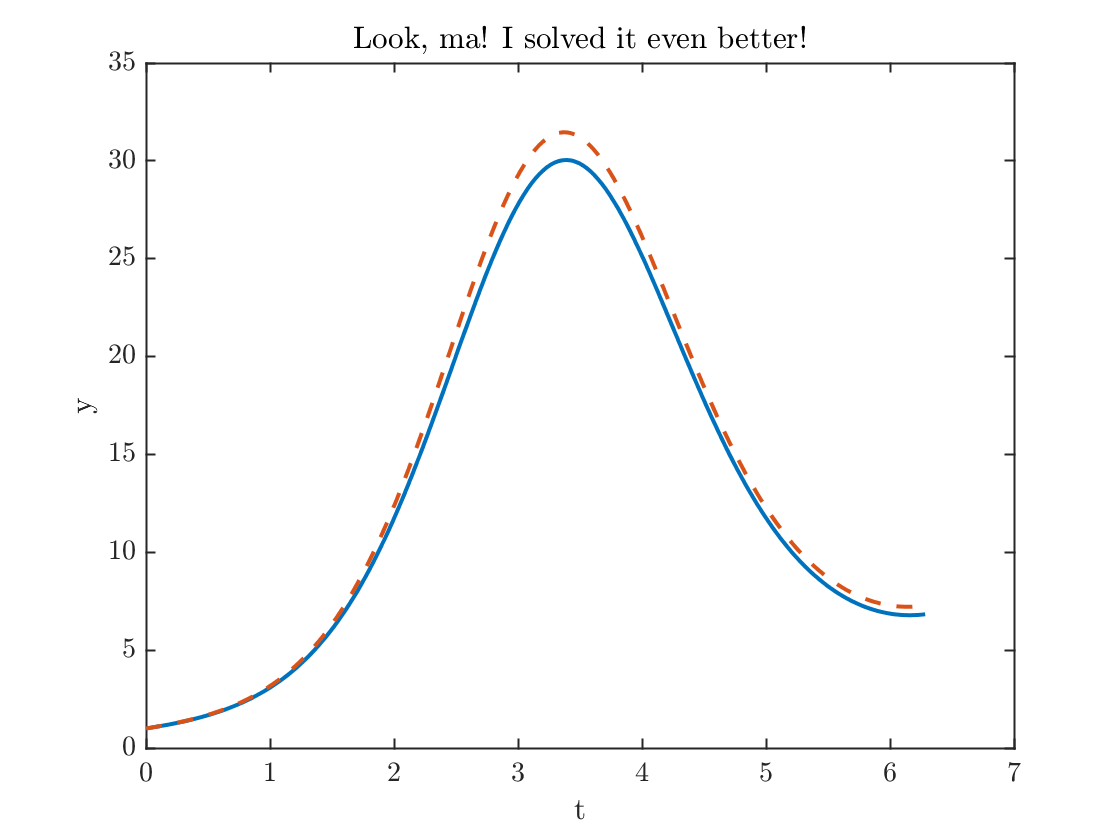error200= max(abs(x-xExact));
fprintf('The maximum error = %0.2f.\n',error200)
The maximum error = 1.49.
fprintf('The maximum error went down by a factor of %f.\n',error100/error200);
The maximum error went down by a factor of 1.907074.

## Using a function

Note how the differential equation is hardcoded inside the for-loop. This is very bad programming practice (can you see why?), but I did it to make things simpler in the demonstration. It is much better practic to define the differential equation inside a function. At the bottom of this file, you will find the definition of a function f. Below I solve the same differential equation again, replacing the explicit formula by a call to the function f. You should do it this way instead.
z=zeros(1,N+1);
z(1)=1;
for n=1:N
z(n+1) = z(n) + h* f(t(n),x(n));
end
plot(t,z)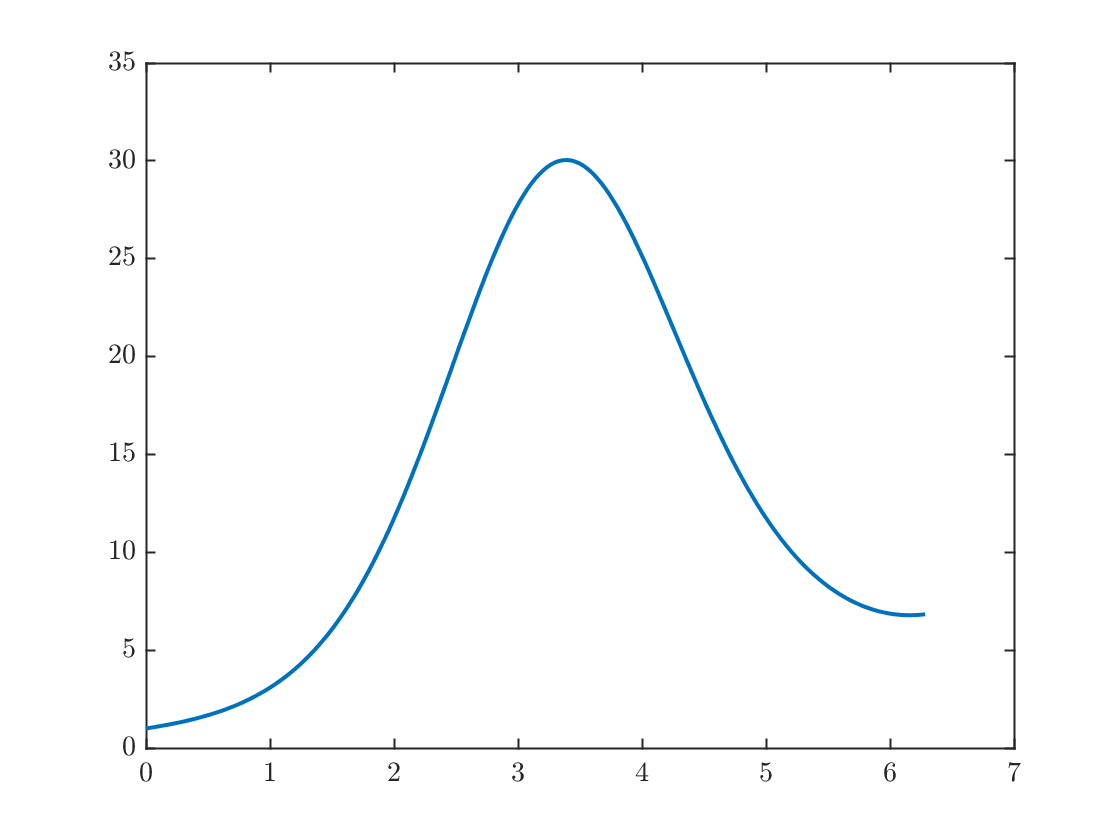One of the real power of numerical methods is that it allows you to see what the solution looks like, even if you can't solve it exactly. The problem below is an example of a Bernoulli differential equation. These can be solved but require more advanced methods.
Question 1: Apply the Euler method to solvefrom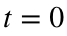to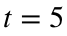. Your code should use a function, just as in the code I used to find z(t) in the most recent section.
Modify the above code (using a function f) so that it solves this ODE
Note that this problem has exact solution.
Then do the following:
• Figure out what is the minimum value of N that you need such that the maximum error is smaller than 0.01. Do this by experimenting with N until you get a small enough error.
• Plot the computed and exact solution using this value of N.
• Show the method is approximately first order by doubling N and computing the ratio of the errors. Doing so may require a much larger value of N, especially in the two further questions below.
• You should find that the solution has exactly one local maximum.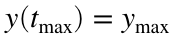. Use the max function to find approximations to both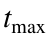and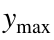. To do this, you need to call the function with two output arguments. Look at the help for the function max by typing doc max at the Matlab >> prompt. Print out these values and, if possible, plot this point with a marker on your plot of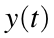.
You should hand in a printout of your code, any figures you print, and a report of a few sentences answering all the questions.
Question 2 The Euler method is an example of a first order method. This means that the error is proportional to h, so that doubling the number of points roughly cuts the error roughly by a factor of two.
The improved Euler method is what's known as a two-step method. It requires two evaluations of the function per time step. At each step, we define two extra quantities before computing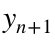.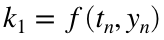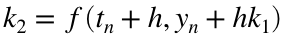The payoff for using this more complicated method is that it is second order! This means that each time you double the number of time steps the error goes down by a factor of four. This is a big deal.
Program this method to solve the same problem from Question 1 and
• Determine how large N needs to be get a maximum error error less than 0.01.
• Show the method is second order by doubling N and seeing that the error has gone down by a factor of four.
• Here we see the payoff of defining f(t,y) in a separate function.
The standard method most people use for general purposes is called fourth-order Rung-Kutta. It uses four evaluations at each step, and the method's total error scales like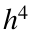For certain applications, such as computing spacecraft trajectories, scientists are likely to use methods that are of much higher order, even 12th order!
function yprime=f(t,y)
yprime = (sin(t)+1/(1+t))*y;
end